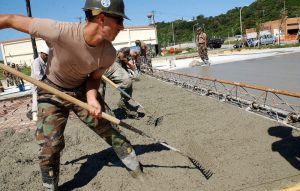Chemistry

# Non-Newtonian fluid

Many people have heard of Isaac Newton at some point. This famous scientist was characterized by developing many scientific theories in the area of mathematics and physics. Isaac Newton described how normal liquids or fluids behave and also made a series of observations of flows that have a viscosity or a constant flow. This means that the flow or viscosity behavior only changes with changes in temperature or pressure. Normally, liquids take the form of the container where they are poured and are known as Newtonian fluids or normal liquids. But not all fluids we observe follow this rule. We then call these strange liquids or non-Newtonian fluids.Related topics

Newtonian fluid

## What is a non-Newtonian fluid?

A non-Newtonian fluid is a homogeneous composition substance that undergoes continuous deformations in the time in which a tension or force is applied to it, regardless of the magnitude of the same, it is a substance that does not have its own shape and that adopts the shape of the container that contains it.

Non-Newtonian fluids change their viscosity or flow behavior when under stress. If a force is applied to these fluids, for example, if you hit them, shake them or jump on them, the sudden application of this type of force or stress can cause them to become thicker and act like a solid, or in some cases result in opposite behavior and may become faster than they were before. As soon as the tension that has been exerted on them is removed, the fluids will return to their previous state.

## Characteristics of non-Newtonian fluid

The main characteristics of a non-Newtonian fluid are as follows.

• It is a substance of homogeneous
• It has resistance to flowing.
• The liquids have the ability to vary depending on the tension
• Their viscosity value is not defined or constant.
• When these liquids are at rest they behave like a liquid and when a force is applied, they increase their viscosity.
• The viscosity of non-Newtonian fluids is totally time-dependent.

## Uses of non-Newtonian fluids

It has been proposed the use of non-Newtonian fluids to fill the potholes that we can find on the roads and thus solve the problem partially. This is because these substances have the peculiarity and capacity to vary their viscosity depending on the different conditions to which they are exposed, such as temperature or stress to which they are submitted. One of the advantages of this idea is that it is possible to cover potholes with a quick action, because the fluid simply has to be emptied into the hole to cover it.

Kevlar has been the primary choice for creating bulletproof vests over the last few decades, but that may come to an end, as it has been shown that this protection would have more effect if done with non-Newtonian bulletproof fluids. This is because non-Newtonian fluids have the amazing ability to change their viscosity depending on the surface tension applied to them.

## Classification of non-Newtonian fluids

Non-Newtonian fluids independent of time: This type of non-Newtonian fluid is subdivided into two groups: those with a threshold and those without. When we speak of threshold, we are referring to the minimum need of shear stress value that these fluids have in order to be able to get into motion, therefore, it has a viscosity that does not vary with time at any shear stress. Non-Newtonian fluids without threshold stress are divided into several groups: pseudoplastics that are characterized by a viscosity that decreases with the increase of the deformation speed so that their shear stress will also be affected; dilatants that are suspensions in which viscosity increases with the increase of the deformation speed that they have by stress. Non-Newtonian fluids with threshold stress can also be of different kinds, for example, ideal plastics that are characterized mainly because they remain in a rigid or solid form until the deformation stress is exceeded. We also find thixotropic and rheopectic fluids.

## Viscosity of non-Newtonian fluid

The viscosity of non-Newtonian fluids is the type of viscosity that can vary with temperature and pressure but does not do so by varying speed.

## Temperature

In non-Newtonian fluids, temperature is responsible for variations in the viscosity of the fluids.

## How to make a non-Newtonian fluid

A simple, non-toxic example can be made when we add a cup of cornstarch to a cup of water. The cornstarch should be added little by little in the water, in small proportions and is mixed slowly. When the mixture approaches the critical concentration typical of a non-Newtonian fluid is when the properties of this fluid become evident. The application of a force by means of a spoon makes the fluid behave more like a solid than a liquid. If left to rest, it recovers its behavior as a liquid.

## Examples

• Soaps and toothpaste.
• Foods such as butter, cheese, jam, tomato sauce, mayonnaise, chewable caramel and yogurt.
• Substances that can be found in nature such as magma and lava.
• Biological fluids such as blood, saliva, mucous membrane and synovial fluid.
• Mud and cement.
Written by Gabriela Briceño V.